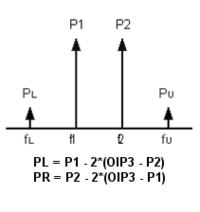# IP3 - Unequal input tones vs Equal input tones

Status
Not open for further replies.

#### passerby

##### Member level 3For 2-tone IP3 measurement, do unequal input tones test get the same result as equal input tones test?

Thanks.

#### vfoneNo, they don't get the same results.
For example for equal input level tones you get 3dB variation of the intermod's amplitude for each 1dB variation of the two-tones level.
When the two-tones are not equal, for example if the left tone is 1dB lower than the right tone, you get 1dB variation on the left intermod, and 2dB variation on the right intermod.where P1 and P2 are the two-tones levels, PL is left intermod level, and PR is the right intermod level, all in dBm.
You have to calculate first the OPI3 of the device.

•diego.fan

Points: 2